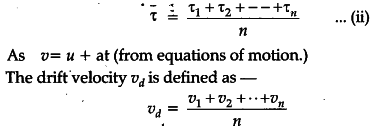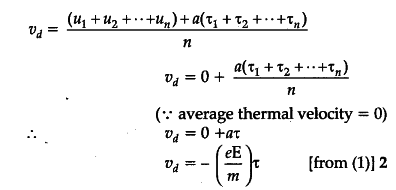# Derive an expression for drift velocity of free electrons

(i) Derive an expression for drift velocity of free electrons.
(ii) How does drift velocity of electrons in a metallic conductor vary with increase in temperature ? Explain.

(i) When conductor is subjected to an electric field E, each electron experience a force.
F=-eE
and acquires an acceleration
a = F/m = - eE/m -----(i)
Here m = mass of election, e = charge, E = electric field.
The average time difference between two consecutive collisions is known as relaxation time of election.(ii) According to drift velocity expression, relaxation time is the time interval between successive collisions of an electron on increasing temperature, the electrons move faster and more collisions occur quickly. Hence, relaxation time decreases with increase in temperature which implies that drift velocity also decreases with temperature.

1 Like